Courses

# Transistor Current Component Electrical Engineering (EE) Notes | EduRev

## Electronic Devices

Created by: Cstoppers Instructors

## Electrical Engineering (EE) : Transistor Current Component Electrical Engineering (EE) Notes | EduRev

The document Transistor Current Component Electrical Engineering (EE) Notes | EduRev is a part of the Electrical Engineering (EE) Course Electronic Devices.
All you need of Electrical Engineering (EE) at this link: Electrical Engineering (EE)

TRANSISTOR CURRENT COMPONENTS: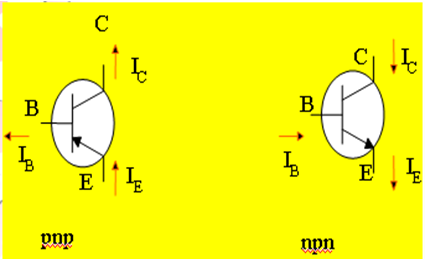FIG 2

The above fig 2 shows the various current components, which flow across the forward biased emitter junction and reverse- biased collector junction. The emitter current Iconsists of hole current IPE (holes crossing from emitter into base) and electron current InE (electrons crossing from base into emitter).The ratio of hole to electron currents, IpE / InE   , crossing the emitter junction is proportional to the  ratio of the conductivity of the p material to that of the n material. In a transistor, the doping of the emitter is made much larger than the doping of the base. This feature ensures (in p-n-p transistor) that the emitter current consists of holes in a larger proportion. Such a situation is desired since the current which results from electrons crossing the emitter junction from base to emitter does not contribute carriers, which can reach the collector.

Not all the holes crossing the emitter junction Jreach the the collector junction Jbecause some of them combine with the electrons in n-type base. If IpC   is hole current at junction Jthere must be a bulk recombination current ( IpE- IpC ) leaving the base.

Actually, electrons enter the base region through the base lead to supply those charges, which have been lost by recombination with the holes injected into the base across JE. If the emitter was open circuited so that IE=0 then IpC would be zero. Under these circumstances, the base current IB and collector current IC would equal the reverse saturation current ICO. If IE≠0 then

IC= ICO- IpC

For a p-n-p transistor, ICO consists of holes moving across Jfrom left to right (base to collector) and electrons crossing JC   in opposite direction, assuming reference direction for ICO,  i.e. from right to left, then for a p-n-p transistor, ICO is negative. For a n-p-n transistor, ICO  is positive.The basic operation will be described using the pnp transistor. The operation of the npn transistor is exactly the same if the roles played by the electron and hole are interchanged.

One p-n junction of a transistor is reverse-biased, whereas the other is forward-biased.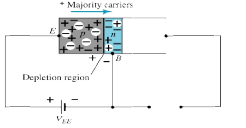Forward-biased junction of a pnp transistor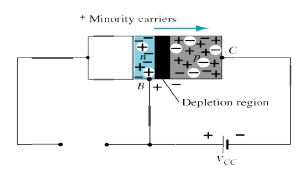Reverse-biased junction of a pnp transistor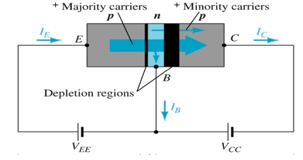Both biasing potentials have been applied to a pnp transistor and resulting majority and minority carrier flows indicated.

Majority carriers (+) will diffuse across the forward-biased p-n junction into the n-type material.

A very small number of carriers (+) will through n-type material to the base terminal. Resulting IB , typically in order of microamperes.

The large number of majority charge carriers will diffuse across the reverse-biased junction into the p-type material connected to the collector terminal.

Applying KCL to the transistor :

IE = IC + IB

IC comprises of two components – the majority and minority carriers

IC = ICmajority + ICOminority

ICO – IC current with emitter terminal open and is called leakage current.

Various parameters which relate the current components are given below:

Emitter efficiency: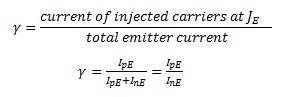Transport Factor: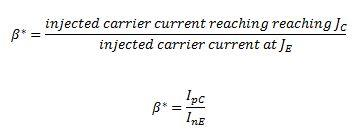Large signal current gain:

The ratio of the negative of collector current increment to the  emitter current change from zero (cut-off)to IE. The large signal current gain of a common base transistor.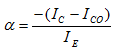Since IC and Ihave opposite signs, then α, as defined, is always positive. Typically numerical values of α lies in the range of 0.90 to 0.995.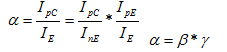The transistor alpha is the product of the transport factor and the emitter efficiency. This statement assumes that the collector multiplication ratio α is unity. α is the ratio of total current crossing JC to hole arriving at the junction.

Offer running on EduRev: Apply code STAYHOME200 to get INR 200 off on our premium plan EduRev Infinity!

,

,

,

,

,

,

,

,

,

,

,

,

,

,

,

,

,

,

,

,

,

;